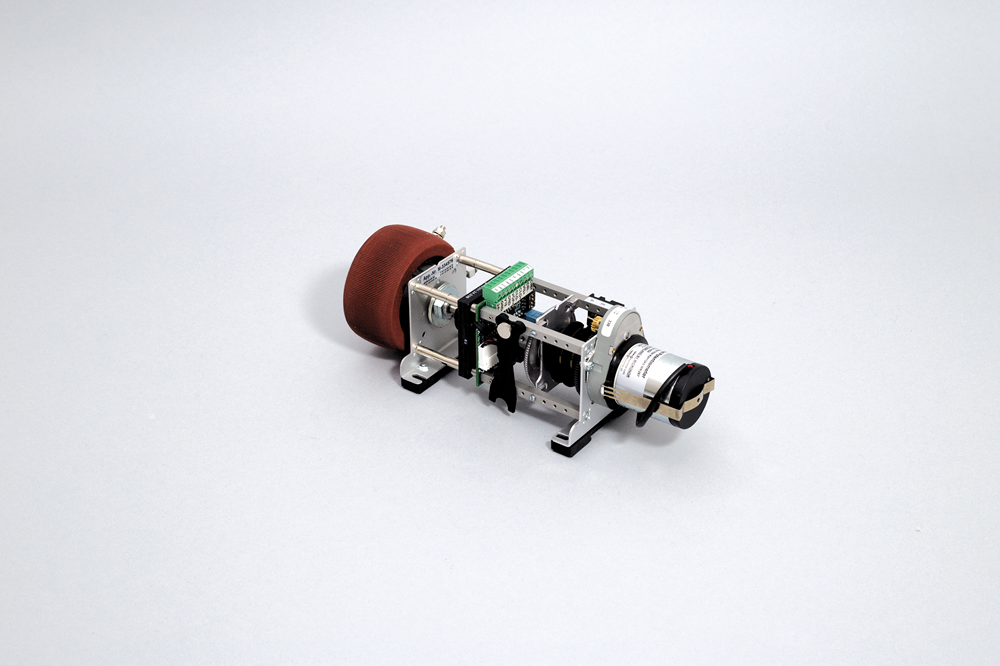# MR266 PLC-Compatible Motorized Potentiometer/Rheostat (20W to 1000W)## MR266

### Features

• DIN rail mount
• Standard non-isolated or special isolated 1kV) interface option
• 24 VDC operation
• Timing options: 10s to 120s
• Quick connections via PHOENIX CombiCon wiring plug
• For applications >600 Watts, contact Micronor sales at +1-805-389-6600 for assistance
• For applications <5 Watts, go to MR267 series

#### TO ORDER

Call +1-805-389-6600 or email sales@micronor.com

### Ordering Information

 MR266-XX-Y-Z   Example: MR266-22-C-12 R=1K Ohms/170 Watts, Isolated 4-20mA Input Control and Isolated 4-20mA Position Feedback where XX=Control Option 10=Non-isolated 4-20mA INPUT Only22=Isolated 4-20mA INPUT/OUTPUT44=Isolated 0-10V INPUT/OUTPUT where Y=Wattage Option A=20 WB=60 WC=170 WD=300 WE=600 W where Z=Resistance (in Ohms) 1=2Ω2=3Ω3=5Ω4=10Ω5=20Ω6=25Ω7=50Ω8=100Ω9=200Ω10=250Ω11=500Ω12=1KΩ13=2KΩ14=2.5KΩ15=5KΩ16=10KΩ17=15KΩ18=20KΩ19=25KΩ20=50Ω NOTE: Not all resistance values may be available in all wattage ratings.

### Related Products